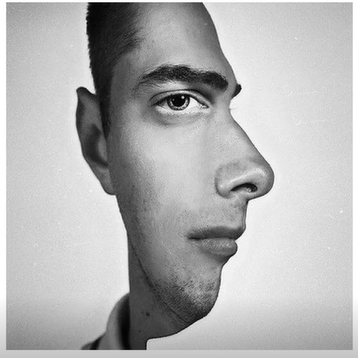# What is a Convolutional Neural Network (CNN)?

Original article was published by Manik Soni on Artificial Intelligence on Medium# Goals and Objective :

1. How does the convolutional neural network work?
2. Convolution Neural Network(CNN) Vs Artificial Neural Network(ANN)
3. How the Convolutional Neural Network(CNN) see the world?
4. Steps to Make Convolutional Neural Network(CNN)

Before we dive deep into Convolution Neural Network(CNN) let us first see some of the examples :

Example 1: Below is an image, what inference you can derive from this image?

Is the man looking in front? Or he is looking at the right side?

So, this basically depends upon the view that we are looking either from the front or from the right side, our brain is deriving the essential features that help the brain to do the classification.

Example 2: This is another famous image.

Is the woman looking at the back? Or Is she is an old woman who is looking at the side?

## How does the convolutional neural network work?

So in order to understand this concept in layman’s language, we give the machine an image and the machine will extract some of the essential features from that image and it will start categorizing the names and the one having the highest votes will be selected.

The above image is one of the examples that will tell us that there, is a huge probability that the image is of ‘cheetah’ so you can see that in order to understand the image, the machine needs to extract important features to do the categorization.

Similarly, you can see this image.

In the above image, the machine will extract some essential features in order to do the categorization for ‘bullet train’.

But, some of the images will make it difficult for a machine to extract features that are similar to the exact image. For example,

The above image is of hand glass but there is a high probability that it is more likely the image is of scissors, not a hand glass.

## Convolution Neural Network(CNN) Vs Artificial Neural Network(ANN)

A research was made by Google and graphically you can see Convolution Neural Network(CNN) is gaining more popularity rather than Artificial Neural Network(ANN).

## Applications of Convolution Neural Network(CNN)

1. Convolutional Neural Network(CNN) for face recognition :

2. Convolutional Neural Network(CNN) for text classification :

3. Convolutional Neural Network(CNN) for image classification:

## Convolutional Neural Network(CNN) Architecture

Convolutional Neural Network(CNN) architecture consists of 3 parts:

1. Input Image: The sample image that we give to the machine for doing the classification.
2. CNN: It stands for Convolutional Neural Network. Training of the Neural Network takes place here and the machine will do the categorization of the input image.
3. Output Label: Give the label of the image.

After the training of the Convolutional Neural Network(CNN), let’s take an example to understand the architecture.

Example: If the CNN of emoji recognition is made, then it will do the categorization in this manner:

## How the Convolutional Neural Network(CNN) see the world?

So, the machine will categorize the image into 2 types :

1. Black and White(B/W)
2. Colored

These images are mapped into arrays to do the classification.

Example: You can see how the machine will extract the essential features from the image and maps it to arrays.

## Steps to Make Convolutional Neural Network(CNN)

Step 1: Convolution: It is a mathematical function that helps to map images to extract essential features. Read the article below to get a deep insight, what is Convolution in Convolutional Neural Network(CNN)?.

Step 2: ReLu(Rectified Linear Unit): ReLU is used to increase nonlinearity in our CNN.

Let’s take this image:

Now adding a feature detector to this image, we get

So, you can analyze that there are some abrupt changes in the above image. There are some parts that are dark and then grey or white. So with the help of the ReLU function, we need to break this linearity.

Now, What ReLU Layer does that it removes all the non-negative value and makes the image nonlinear.

Step 3: Max Pooling: Max Pooling helps to reduce the feature map in order to do the classification more precisely. Read the article below to get a deep insight, what is Max Pooling in Convolutional Neural Network(CNN)?.

Step 4:Flattening: In the previous step we have created a pooled feature map.

Now, we need to flatten our pooled feature map into a single feature column.

Because we need to fill up the input layer to Neural Network.

Summary:

Step 5:Full Connection: Now, doing the full connection in order to train our neural network.

Example: Let’s take an example to categorize that the given image is of a dog or of a cat.

So, hidden layer neurons help to do the prediction based upon training.